# NCERT Solutions For Class 10 Maths Chapter 3 Exercise 3.2 – Pair of Linear Equations in two Variables

Download NCERT Solutions For Class 10 Maths Chapter 3 Exercise 3.2 – Pair of Linear Equations in two Variables. This Exercise contains 7 questions, for which detailed answers have been provided in this note. In case you are looking at studying the remaining Exercise for Class 10 for Maths NCERT solutions for other Chapters, you can click the link at the end of this Note.

### NCERT Solutions For Class 10 Maths Chapter 3 Exercise 3.2 – Pair of Linear Equations in two Variables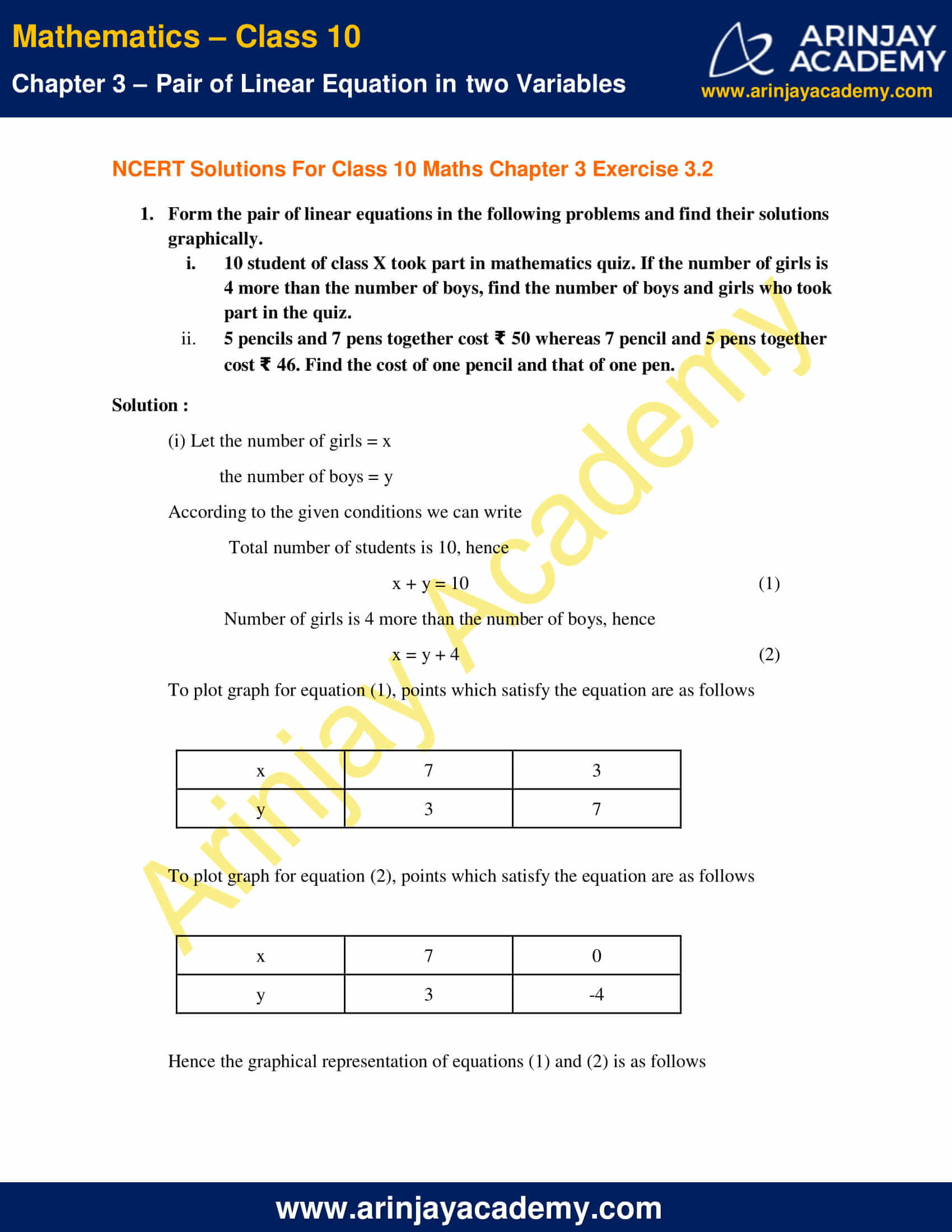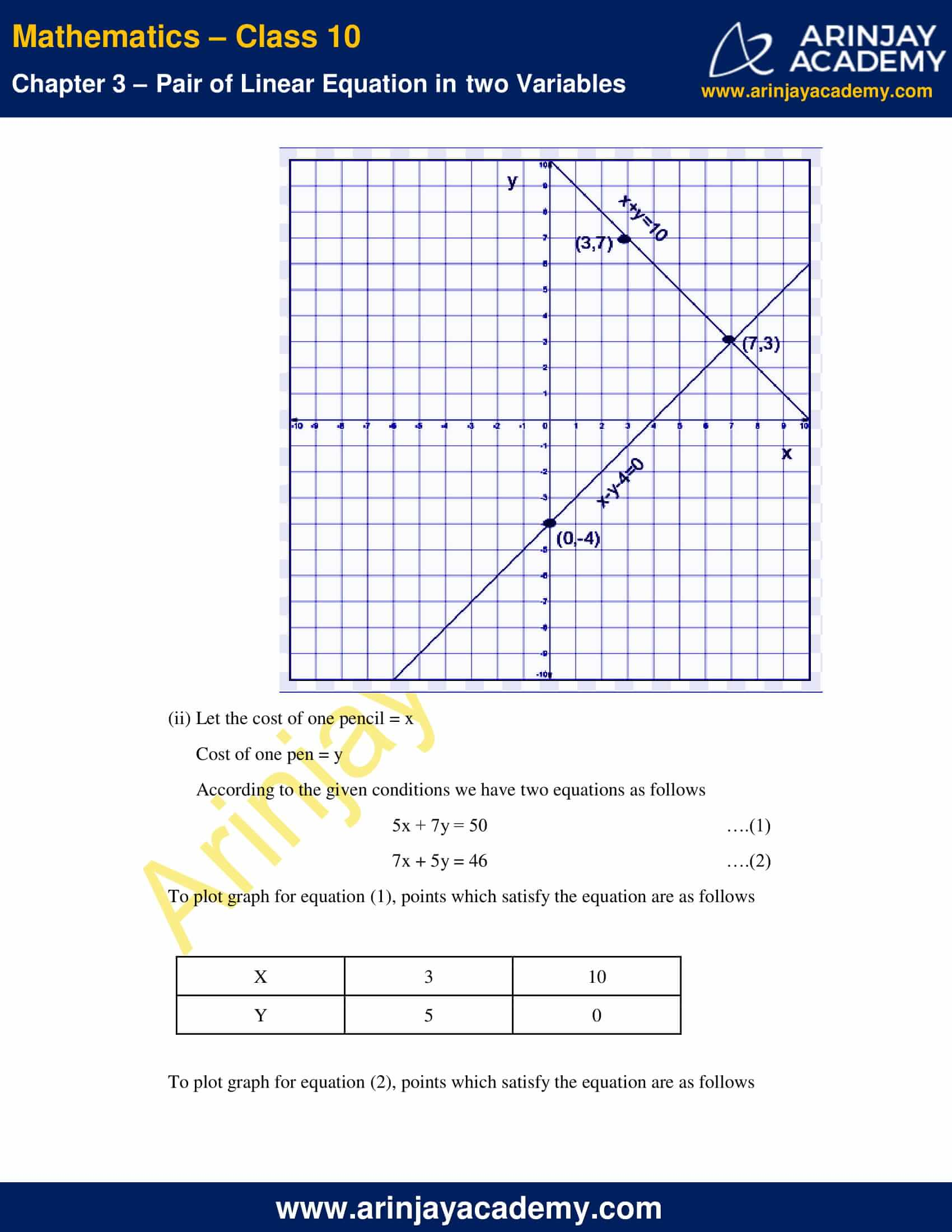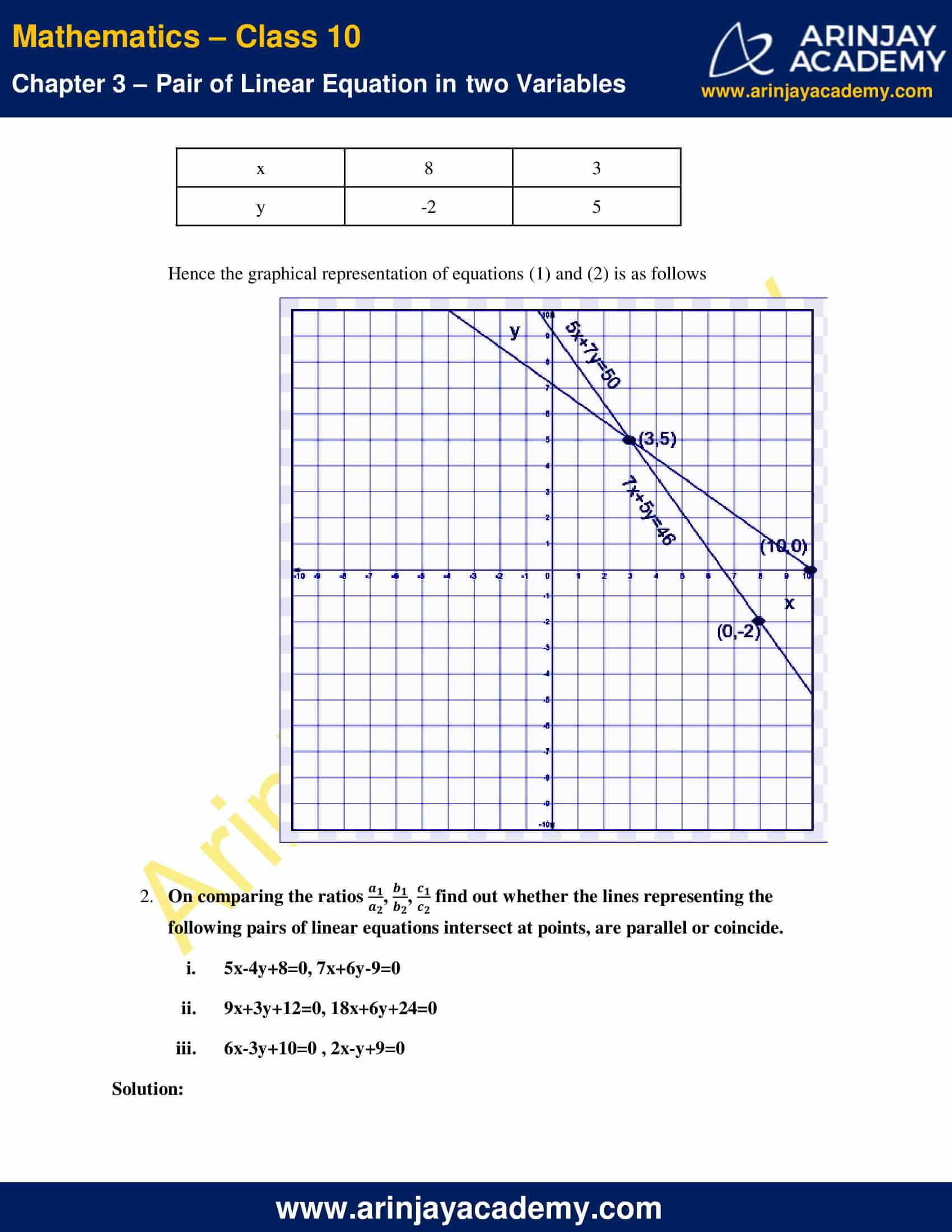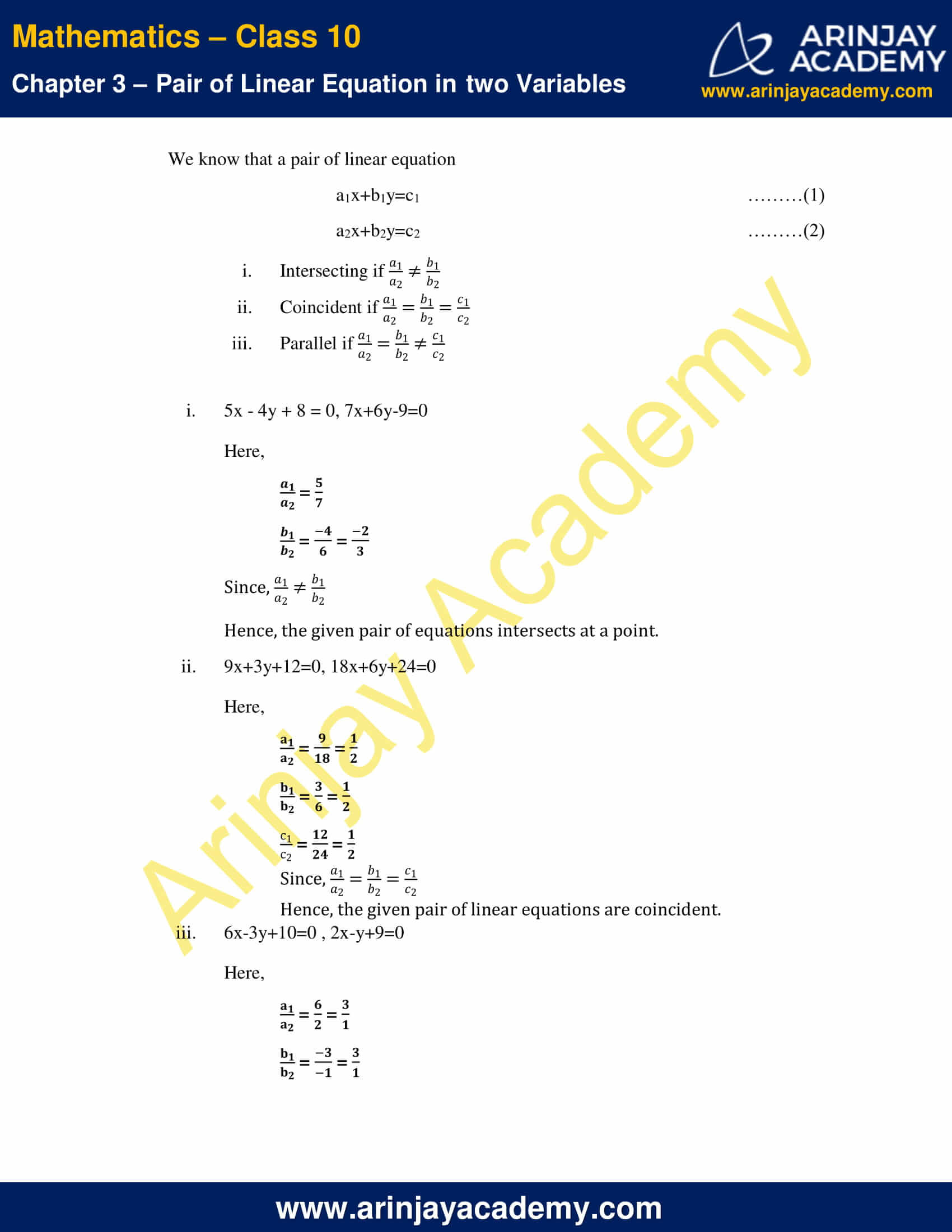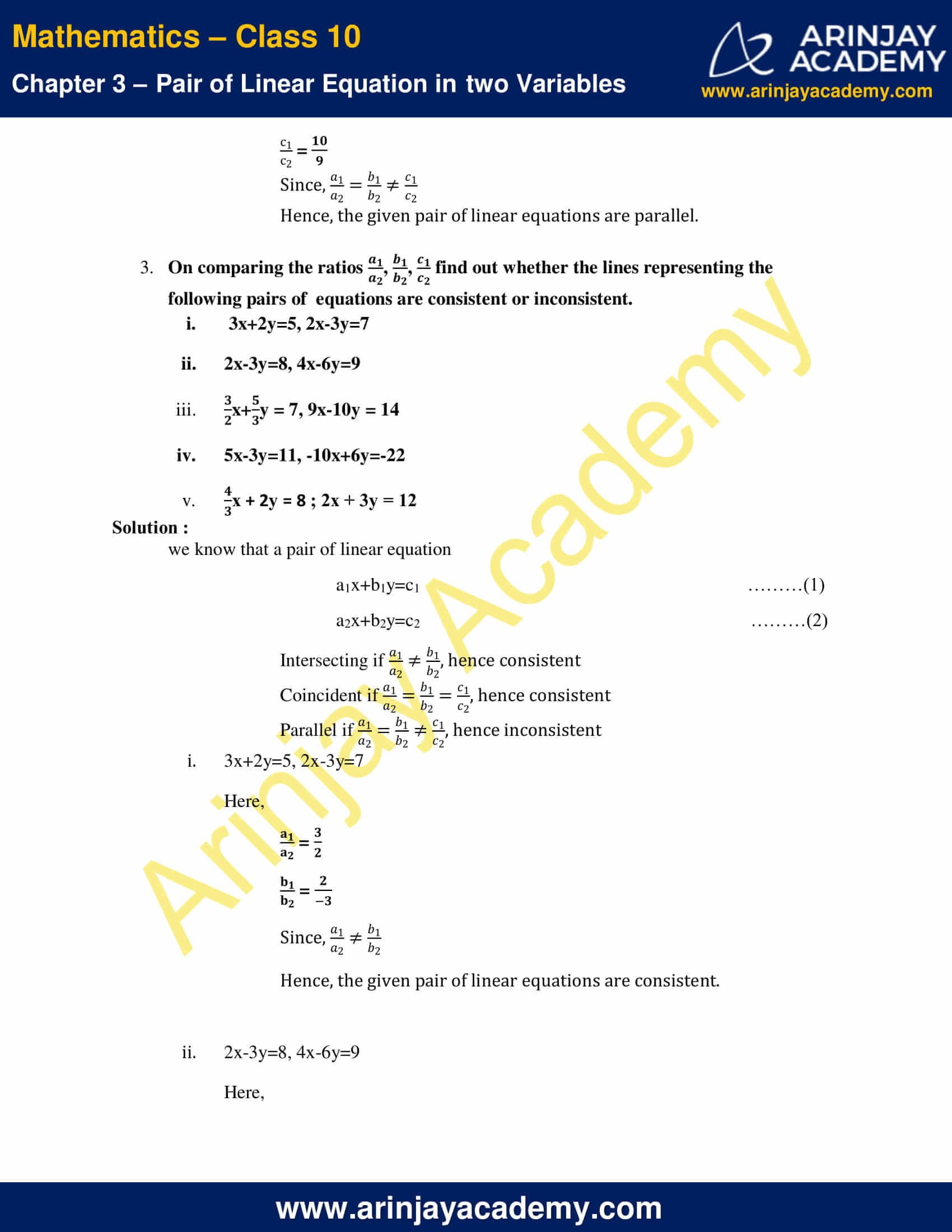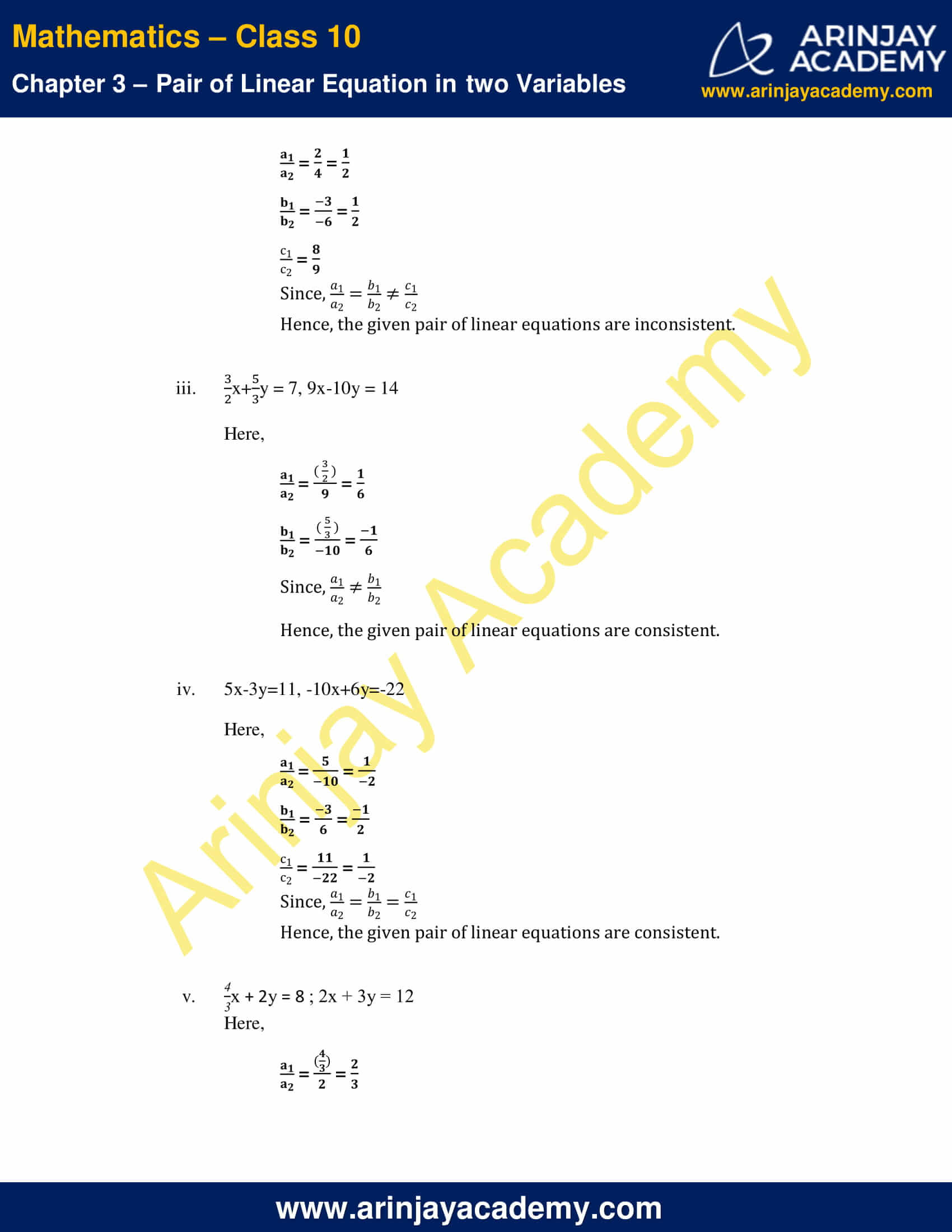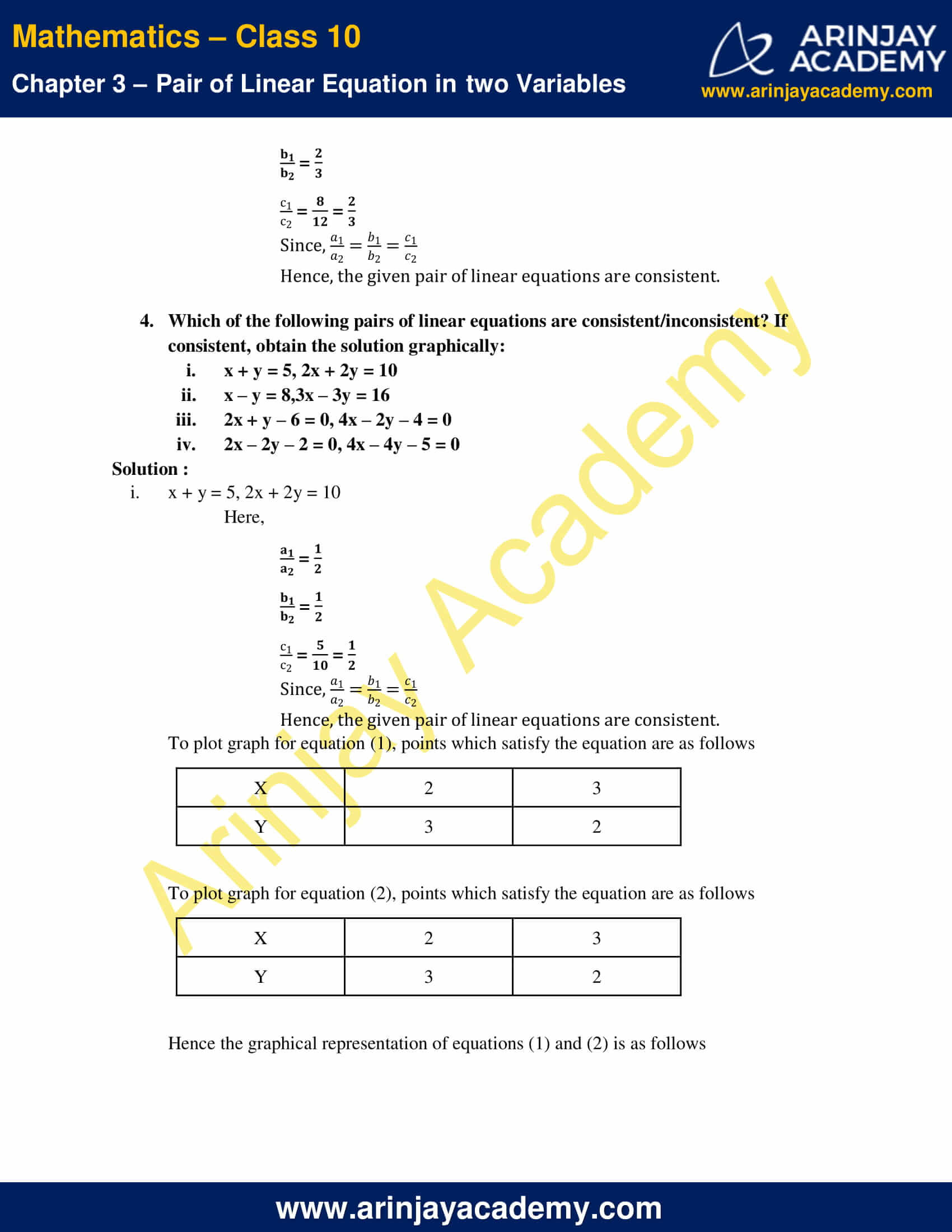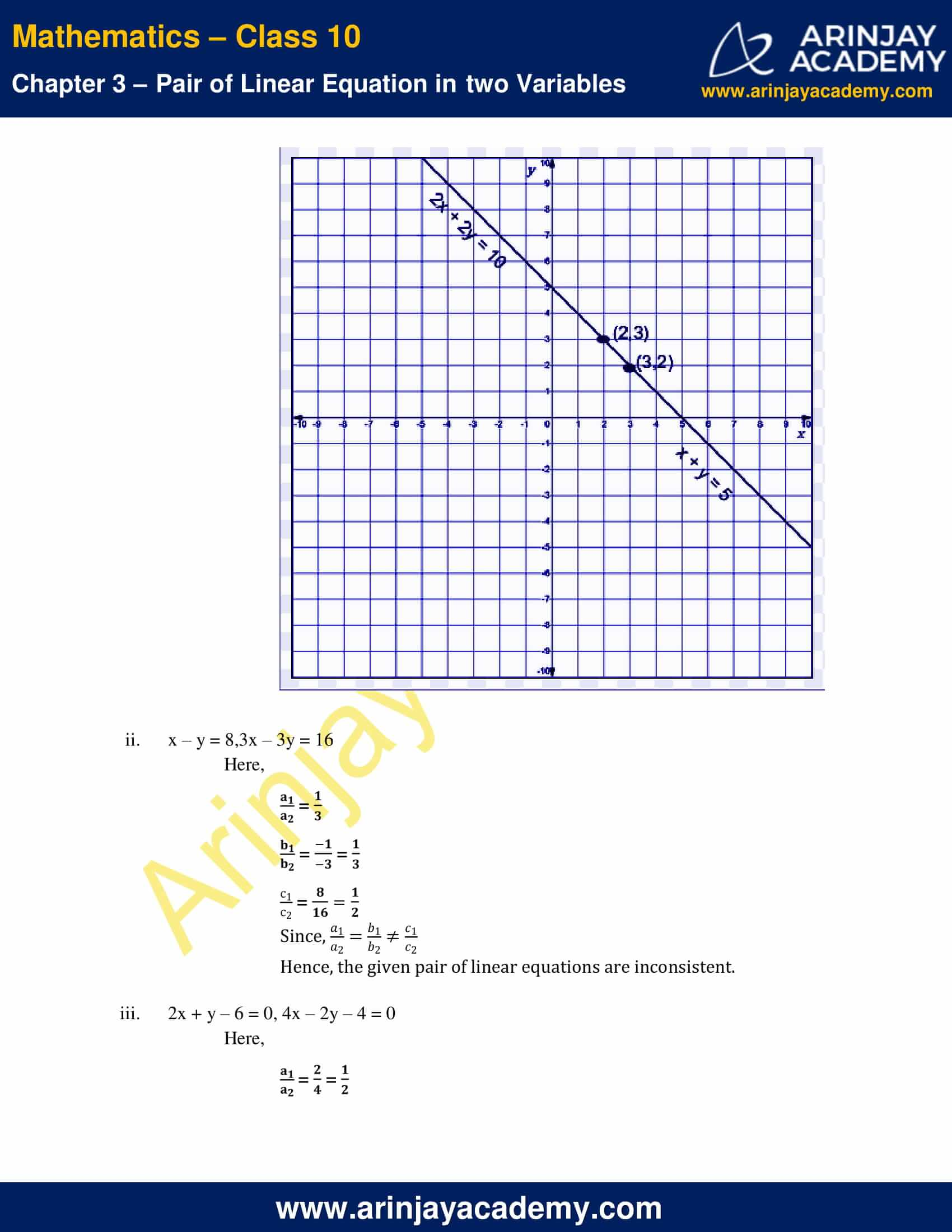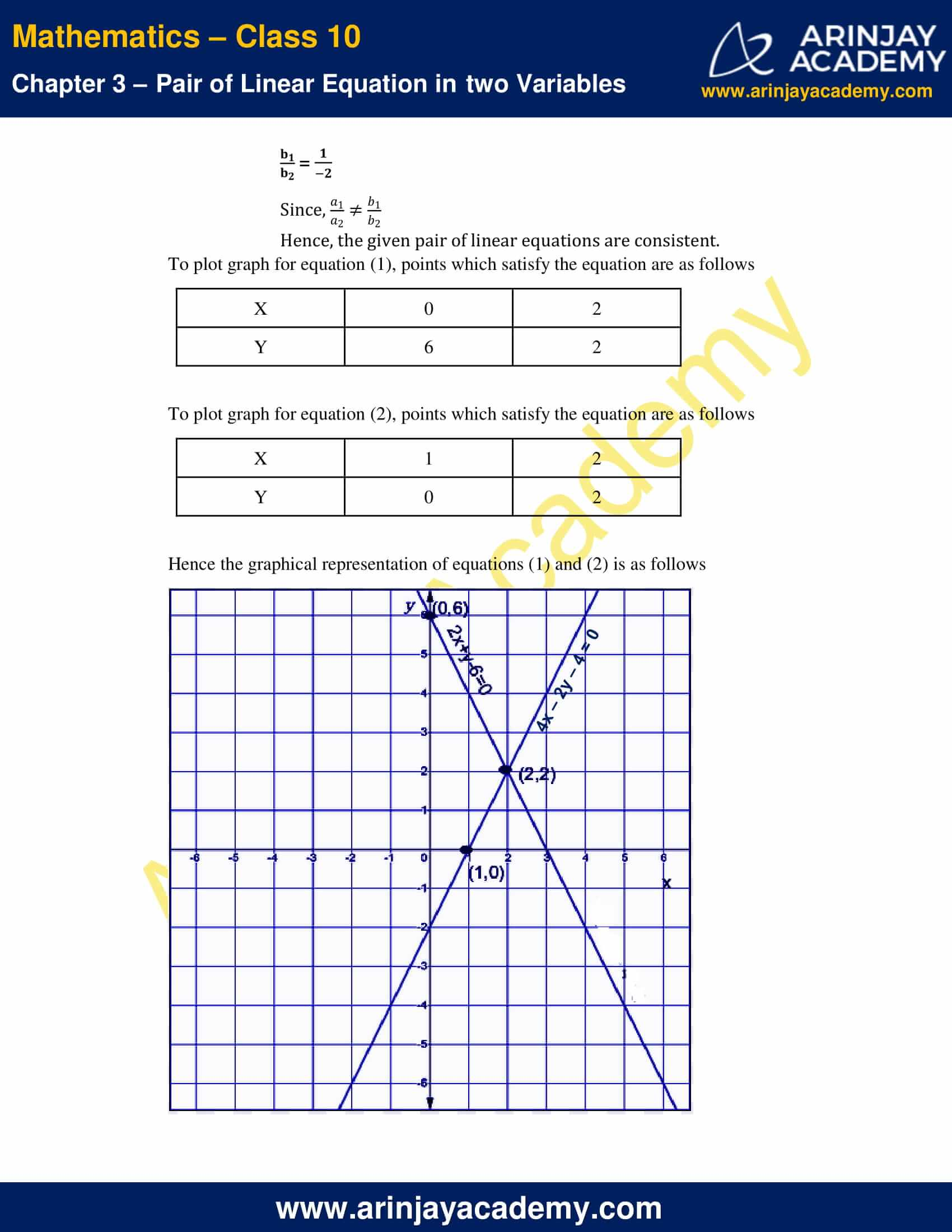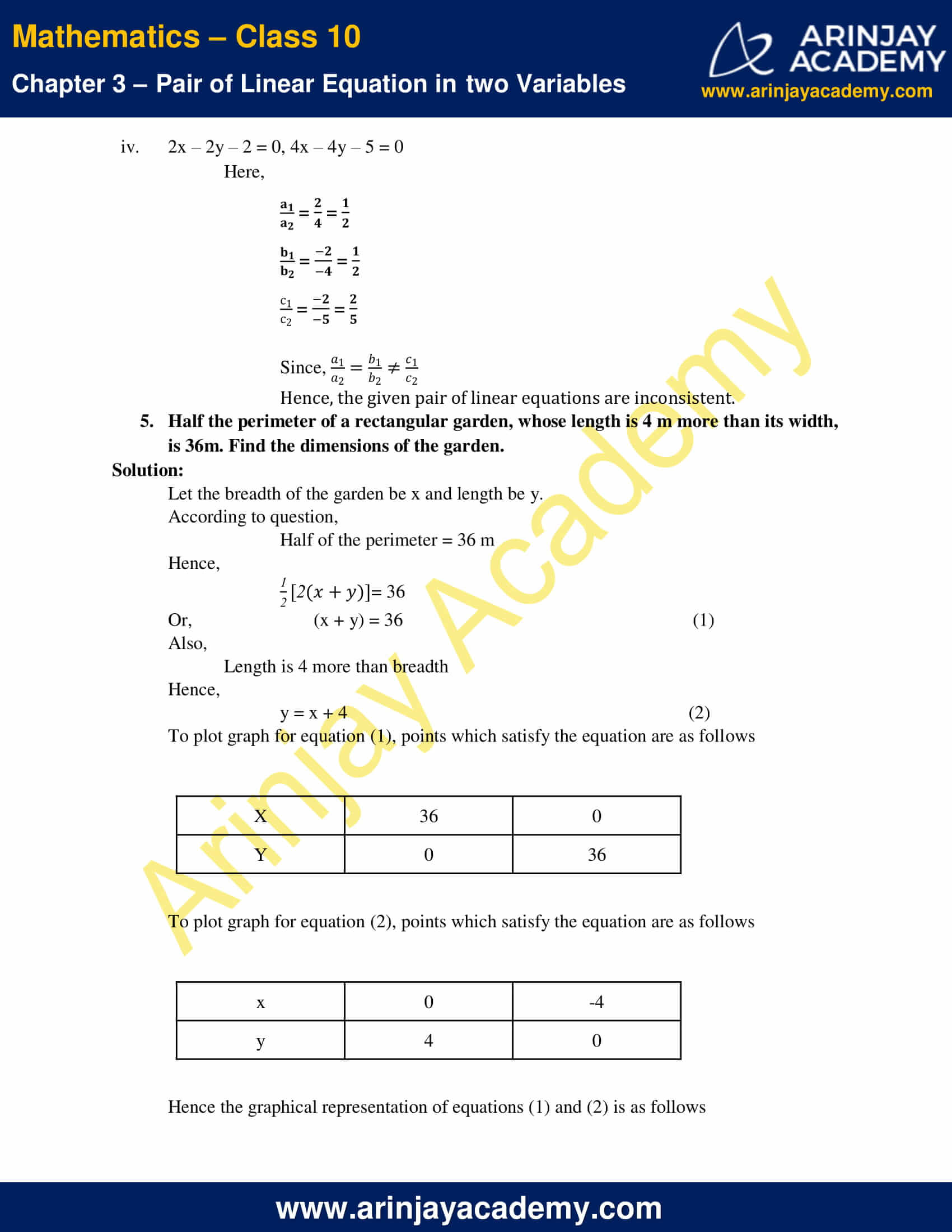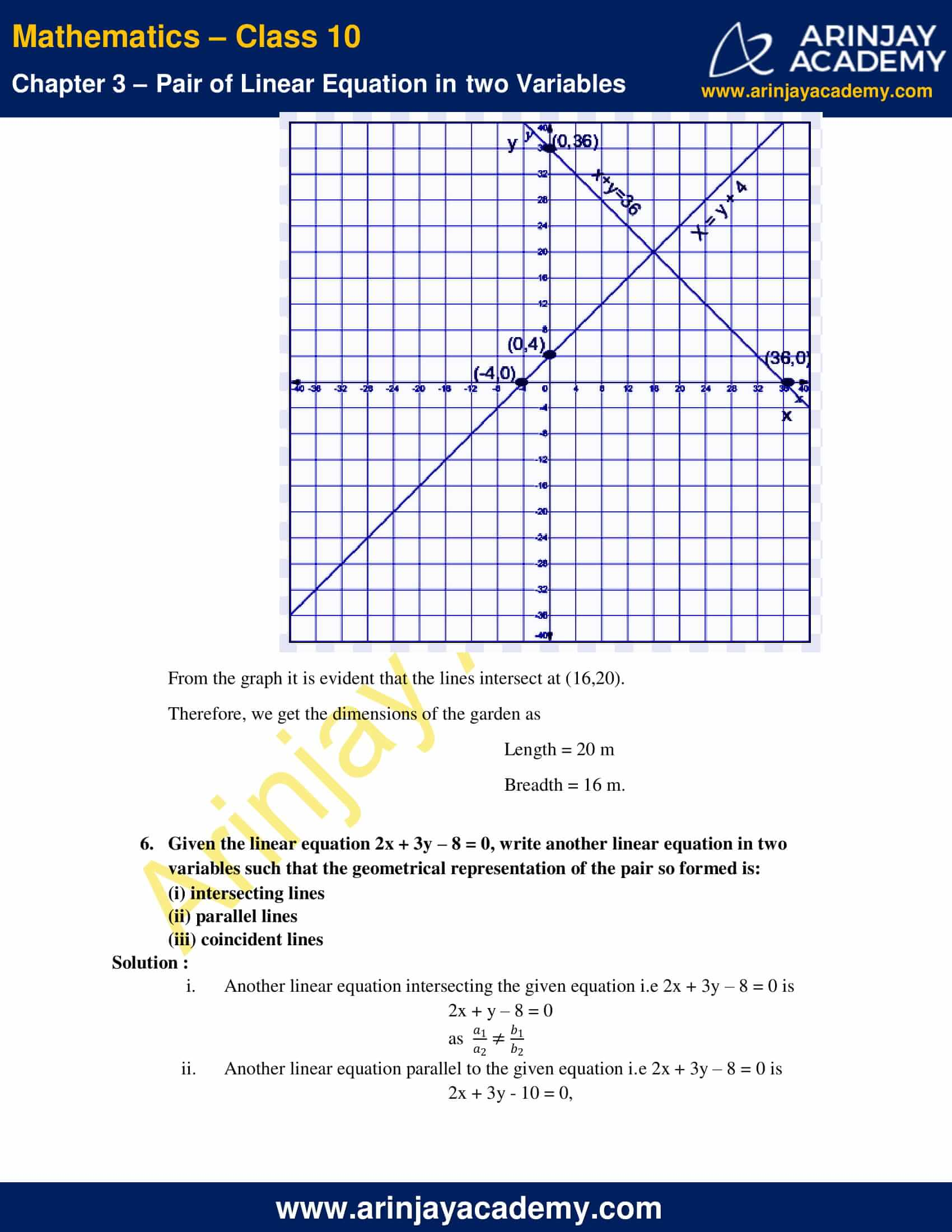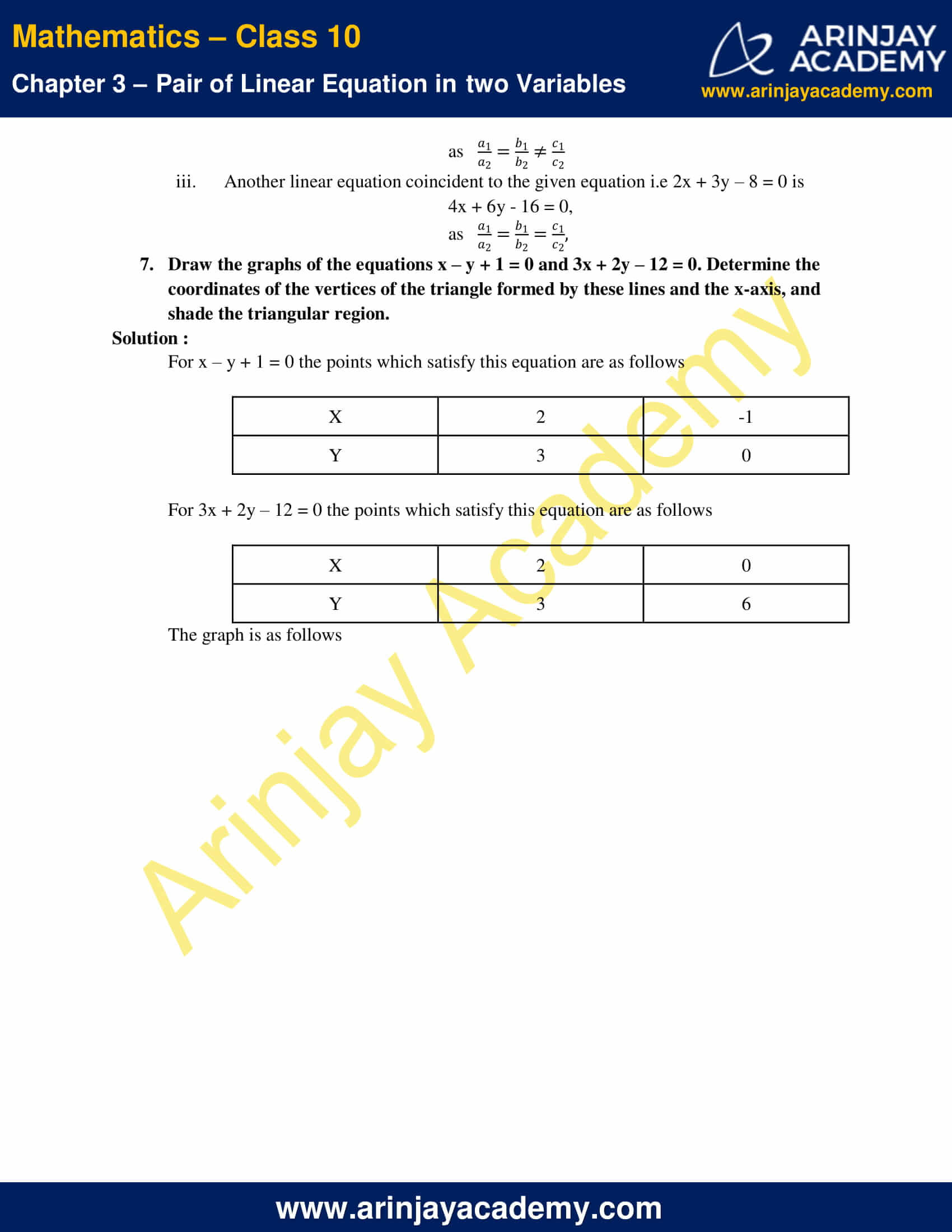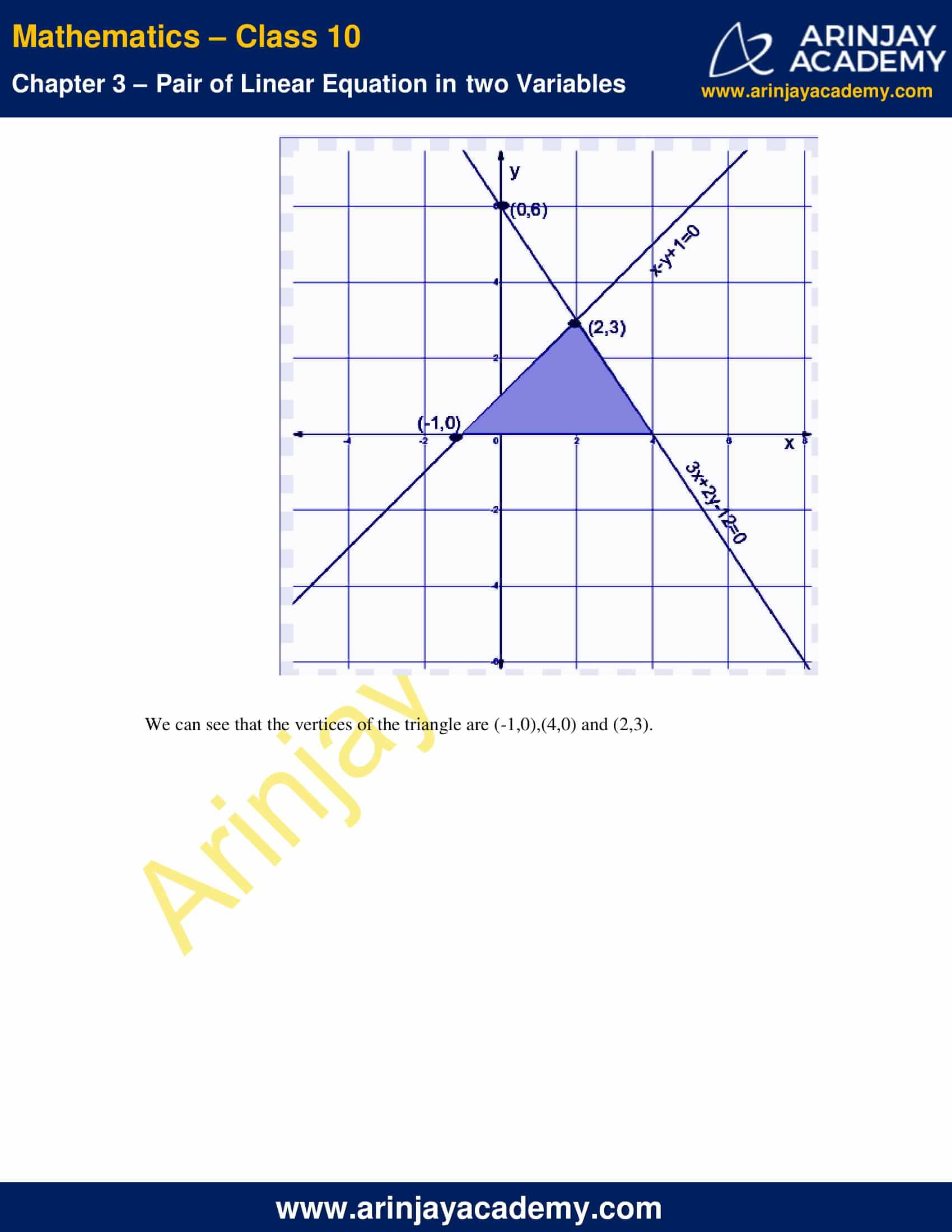NCERT Solutions For Class 10 Maths Chapter 3 Exercise 3.2 – Pair of Linear Equations in two Variables

1. Form the pair of linear equations in the following problems and find their solutions graphically.

i. 10 student of class X took part in mathematics quiz. If the number of girls is 4 more than the number of boys, find the number of boys and girls who took part in the quiz.

ii. 5 pencils and 7 pens together cost 50 whereas 7 pencil and 5 pens together cost 46. Find the cost of one pencil and that of one pen.

Solution :

(i) Let the number of girls = x
the number of boys = y
According to the given conditions we can write
Total number of students is 10, hence
x + y = 10  ..(1)
Number of girls is 4 more than the number of boys, hence
x = y + 4    ..(2)
To plot graph for equation (1), points which satisfy the equation are as follows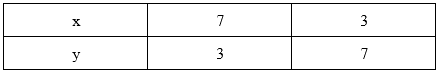To plot graph for equation (2), points which satisfy the equation are as follows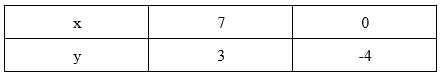Hence the graphical representation of equations (1) and (2) is as follows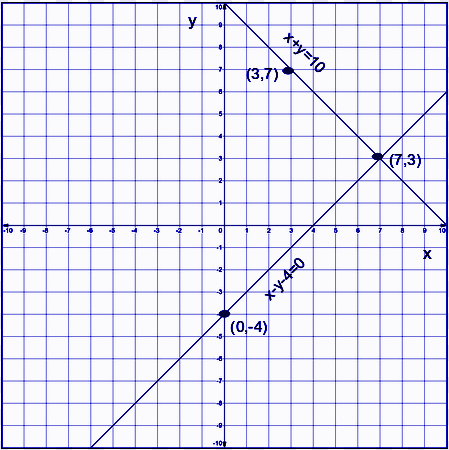(ii) Let the cost of one pencil = x
Cost of one pen = y
According to the given conditions we have two equations as follows
5x + 7y = 50    ….(1)
7x + 5y = 46    ….(2)
To plot graph for equation (1), points which satisfy the equation are as follows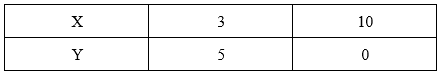To plot graph for equation (2), points which satisfy the equation are as follows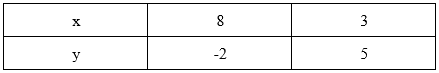Hence the graphical representation of equations (1) and (2) is as follows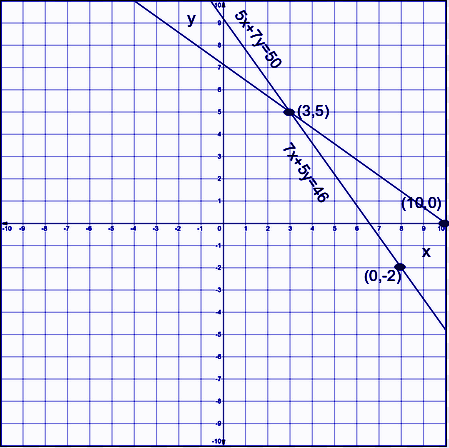2. On comparing the ratios (a1/a2), (b1/b2), (c1/c2), find out whether the lines representing the following pairs of linear equations intersect at points, are parallel or coincide.

i. 5x-4y+8=0, 7x+6y-9=0
ii. 9x+3y+12=0, 18x+6y+24=0
iii. 6x-3y+10=0, 2x-y+9=0

Solution:

We know that a pair of linear equation

a1x+b1y=c1   …(1)
a2x+b2y=c…(2)

i. Intersecting if (a1/a2) ≠ (b1/b2)

ii. Coincident if (a1/a2) = (b1/b2) = (c1, c2)

iii. Parallel if (a1/a2) = (b1/b2) ≠ (c1, c2)

i. 5x – 4y + 8 = 0, 7x+6y-9=0

Here,
(a1/a2)= (5/7)
(b1/b2)= (-4/6) = (-2/3)
Since, (a1/a2) ≠ (b1/b2)
Hence, the given pair of equations intersects at a point.

ii. 9x+3y+12=0, 18x+6y+24=0

Here,
(a1/a2) = 9/18 = 1/2
(b1/b2) = 3/6 = 1/2
(c1/ c2) = 12/24 = 1/2
Since, (a1/a2) =(b1/b2) =(c1/ c2)
Hence, the given pair of linear equations are coincident.

iii. 6x-3y+10=0 , 2x-y+9=0

Here,
(a1/a2) = 6/2 = 3/1
(b1/b2) = (-3/-1) = (3/1)
(c1/ c2) = 10/9
Since, (a1/a2) = (b1/b2) ≠ (c1/ c2)
Hence, the given pair of linear equations are parallel.

3. On comparing the ratios (a1/a2), (b1/b2), (c1, c2). Find out whether the lines representing the following pairs of  equations are consistent or inconsistent.

i. 3x+2y=5, 2x-3y=7
ii. 2x-3y=8, 4x-6y=9
iii. (3/2)x+(5/3)y = 7, 9x-10y = 14
iv. 5x-3y=11, -10x+6y=-22
v. (4/3)x + 2y = 8 ; 2x + 3y = 12

Solution :

we know that a pair of linear equation
a1x+b1y=c1      …(1)
a2x+b2y=c2      …(2)
Intersecting if (a1/a2) ≠ (b1/b2), hence consistent
Coincident if (a1/a2) = (b1/b2) = (c1/ c2), hence consistent
Parallel if (a1/a2) = (b1/b2) ≠ (c1/ c2), hence inconsistent

i. 3x+2y=5, 2x-3y=7

Here,
(a1/a2) = 3/2
(b1/b2) = (2/-3)
Since, (a1/a2) ≠ (b1/b2)
Hence, the given pair of linear equations are consistent.

ii. 2x-3y=8, 4x-6y=9

Here,
(a1/a2) = 2/4 = 1/2
(b1/b2) = (-3/-6) = 1/2
(c1/ c2) = 8/9
Since, (a1/a2) = (b1/b2) ≠ (c1/ c2)
Hence, the given pair of linear equations are inconsistent.

iii. (3/2)x+ (5/3)y = 7, 9x-10y = 14

Here,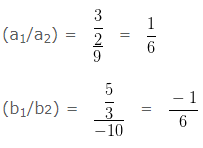Since, (a1/a2) ≠ (b1/b2)
Hence, the given pair of linear equations are consistent.

iv. 5x-3y=11, -10x+6y=-22

Here,
(a1/a2) = (5/-10) = (1/-2)
(b1/b2) = (-3/6) = (-1/2)
(c1/ c2) = (11/-22) = (1/-2)
Since, (a1/a2) = (b1/b2) = (c1/ c2)
Hence, the given pair of linear equations are consistent.

v. (4/3)x + 2y = 8 ; 2x + 3y = 12

Here,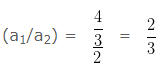(b1/b2) = 2/3
(c1/ c2) = 8/12 = 2/3
Since, (a1/a2) = (b1/b2) =(c1/ c2)
Hence, the given pair of linear equations are consistent.

4. Which of the following pairs of linear equations are consistent/inconsistent? If consistent, obtain the solution graphically:

i. x + y = 5, 2x + 2y = 10
ii. x – y = 8,3x – 3y = 16
iii. 2x + y – 6 = 0, 4x – 2y – 4 = 0
iv. 2x – 2y – 2 = 0, 4x – 4y – 5 = 0

Solution :

i. x + y = 5, 2x + 2y = 10

Here,
(a1/a2) = 1/2
(b1/b2) = 1/2
(c1/ c2) = 5/10 = 1/2
Since, (a1/a2) = (b1/b2) = (c1/ c2)
Hence, the given pair of linear equations are consistent.

To plot graph for equation (1), points which satisfy the equation are as follows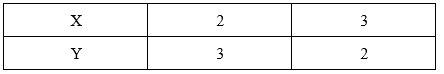To plot graph for equation (2), points which satisfy the equation are as follows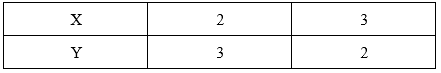Hence the graphical representation of equations (1) and (2) is as follows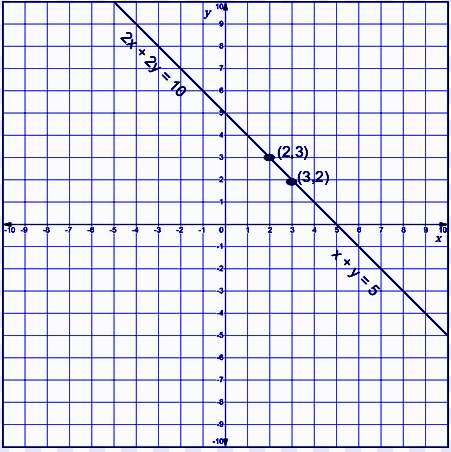ii. x – y = 8,3x – 3y = 16

Here,
(a1/a2) = 1/3
(b1/b2) = (-1/-3) = 1/3
(c1/ c2) = 8/16 = 1/2
Since, (a1/a2) = (b1/b2) ≠ (c1/ c2)
Hence, the given pair of linear equations are inconsistent.

iii. 2x + y – 6 = 0, 4x – 2y – 4 = 0

Here,
(a1/a2) = 2/4 = 1/2
(b1/b2) = (1/-2)
Since, (a1/a2) ≠ (b1/b2)
Hence, the given pair of linear equations are consistent.

To plot graph for equation (1), points which satisfy the equation are as follows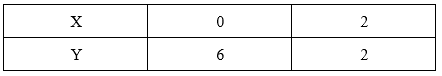To plot graph for equation (2), points which satisfy the equation are as follows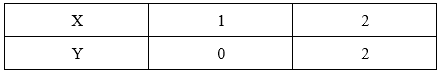Hence the graphical representation of equations (1) and (2) is as follows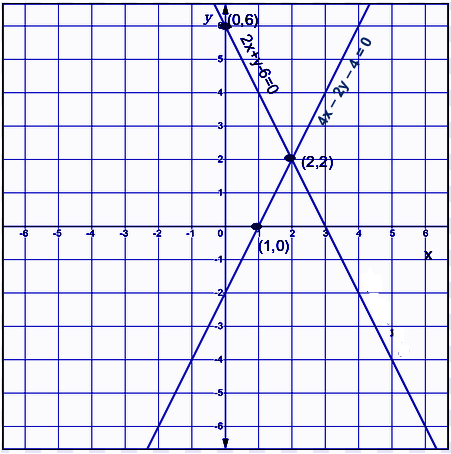iv. 2x – 2y – 2 = 0, 4x – 4y – 5 = 0

Here,
(a1/a2) = 2/4 = 1/2
(b1/b2) = (-2/-4) = 1/2
(c1/ c2) = (-2/-5) = 2/5
Since, (a1/a2) = (b1/b2) ≠ (c1/ c2)
Hence, the given pair of linear equations are inconsistent.

5. Half the perimeter of a rectangular garden, whose length is 4 m more than its width, is 36m. Find the dimensions of the garden.

Solution:

Let the breadth of the garden be x and length be y.
According to question,
Half of the perimeter = 36 m
Hence,
(1/2) [2(x+y)] = 36
Or, (x + y) = 36  ..(1)
Also,
Length is 4 more than breadth
Hence,
y = x + 4   ..(2)
To plot graph for equation (1), points which satisfy the equation are as follows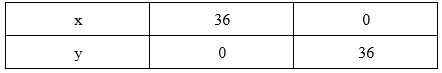To plot graph for equation (2), points which satisfy the equation are as follows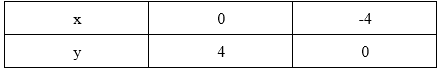Hence the graphical representation of equations (1) and (2) is as follows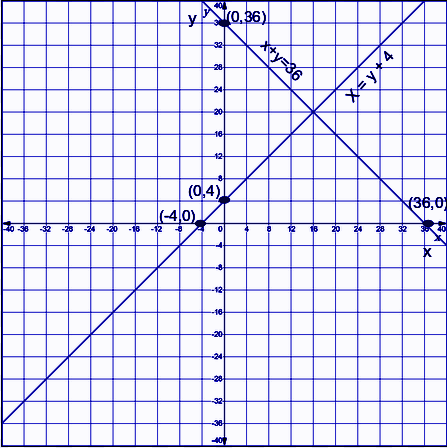From the graph it is evident that the lines intersect at (16,20).
Therefore, we get the dimensions of the garden as
Length = 20 m
Breadth = 16 m.

6. Given the linear equation 2x + 3y – 8 = 0, write another linear equation in two variables such that the geometrical representation of the pair so formed is:
(i) intersecting lines
(ii) parallel lines
(iii) coincident lines

Solution :

i. Another linear equation intersecting the given equation i.e 2x + 3y – 8 = 0 is 2x + y – 8 = 0
as (a1/a2) ≠ (b1/b2)

ii. Another linear equation parallel to the given equation i.e 2x + 3y – 8 = 0 is 2x + 3y – 10 = 0,
as (a1/a2) = (b1/b2) ≠ (c1/ c2)

iii. Another linear equation coincident to the given equation i.e 2x + 3y – 8 = 0 is 4x + 6y – 16 = 0,
as (a1/a2) = (b1/b2) = (c1/ c2)

7. Draw the graphs of the equations x – y + 1 = 0 and 3x + 2y – 12 = 0. Determine the coordinates of the vertices of the triangle formed by these lines and the x-axis, and shade the triangular region.

Solution :

For x – y + 1 = 0 the points which satisfy this equation are as follows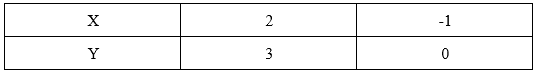For 3x + 2y – 12 = 0 the points which satisfy this equation are as follows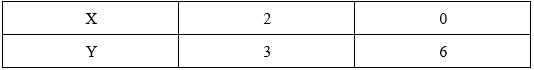The graph is as follows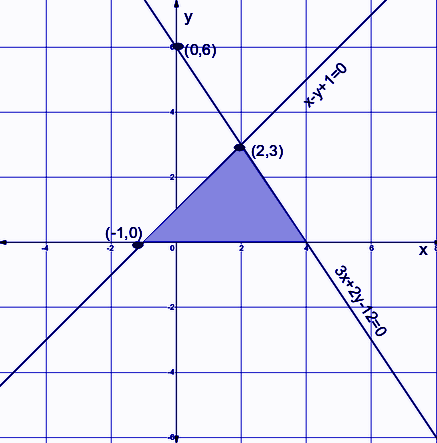We can see that the vertices of the triangle are (-1,0),(4,0) and (2,3).

NCERT Solutions for Class 10 Maths Chapter 3 Exercise 3.2 – Pair of Linear Equations in two Variables, has been designed by the NCERT to test the knowledge of the student on the topic – Graphical Method of Solution of a Pair of Linear Equations

Download NCERT Solutions For Class 10 Maths Chapter 3 Exercise 3.2 –  Pair of Linear Equations in two Variables